Get inspired by the success stories of our students in IIT JAM MS, ISI  MStat, CMI MSc DS.  Learn More

# ISI MStat PSB 2008 Problem 8 | Bivariate Normal Distribution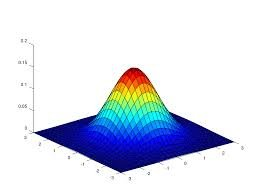This is a very beautiful sample problem from ISI MStat PSB 2008 Problem 8. It's a very simple problem, based on bivariate normal distribution, which again teaches us that observing the right thing makes a seemingly laborious problem beautiful . Fun to think, go for it !!

## Problem- ISI MStat PSB 2008 Problem 8

Let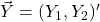have the bivariate normal distribution,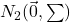,

where,= \begin{pmatrix} \sigma_1^2 & \rho\sigma_1\sigma_2 \\ \rho\sigma_2\sigma_1 & \sigma^2 \end{pmatrix} ;

Obtain the mean ad variance of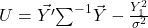.

### Prerequisites

Bivariate Normal

Conditonal Distribution of Normal

Chi-Squared Distribution

## Solution :

This is a very simple and cute problem, all the labour reduces once you see what to need to see !

Remember , the pdf of?

Isn't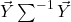is the exponent of e, in the pdf of bivariate normal ?

So, we can say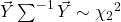. Can We ?? verify it !!

Also, clearly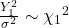; since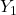follows univariate normal.

So, expectation is easy to find accumulating the above deductions, I'm leaving it as an exercise .

Calculating the variance may be a laborious job at first, but now lets imagine the pdf of the conditional distribution of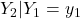, what is the exponent of e in this pdf ??, right !!

and also ,. Now doing the last piece of subtle deduction, and claiming that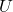and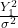are independently distributed . Can you argue why ?? go ahead . So,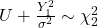.

So,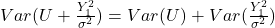, [ since, Variance of a R.V following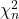is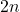.]

Hence the solution concludes.

## Food For Thought

Before leaving, lets broaden our mind and deal with Multivariate Normal !

Let,be a 1x4 random vector, such that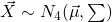,is positive definite matrix, then can you show that,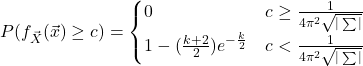Where,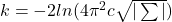.

Keep you thoughts alive !!

## Subscribe to Cheenta at Youtube

This is a very beautiful sample problem from ISI MStat PSB 2008 Problem 8. It's a very simple problem, based on bivariate normal distribution, which again teaches us that observing the right thing makes a seemingly laborious problem beautiful . Fun to think, go for it !!

## Problem- ISI MStat PSB 2008 Problem 8

Lethave the bivariate normal distribution,,

where,= \begin{pmatrix} \sigma_1^2 & \rho\sigma_1\sigma_2 \\ \rho\sigma_2\sigma_1 & \sigma^2 \end{pmatrix} ;

Obtain the mean ad variance of.

### Prerequisites

Bivariate Normal

Conditonal Distribution of Normal

Chi-Squared Distribution

## Solution :

This is a very simple and cute problem, all the labour reduces once you see what to need to see !

Remember , the pdf of?

Isn'tis the exponent of e, in the pdf of bivariate normal ?

So, we can say. Can We ?? verify it !!

Also, clearly; sincefollows univariate normal.

So, expectation is easy to find accumulating the above deductions, I'm leaving it as an exercise .

Calculating the variance may be a laborious job at first, but now lets imagine the pdf of the conditional distribution of, what is the exponent of e in this pdf ??, right !!

and also ,. Now doing the last piece of subtle deduction, and claiming thatandare independently distributed . Can you argue why ?? go ahead . So,.

So,, [ since, Variance of a R.V followingis.]

Hence the solution concludes.

## Food For Thought

Before leaving, lets broaden our mind and deal with Multivariate Normal !

Let,be a 1x4 random vector, such that,is positive definite matrix, then can you show that,Where,.

Keep you thoughts alive !!

## Subscribe to Cheenta at Youtube

This site uses Akismet to reduce spam. Learn how your comment data is processed.

### Knowledge Partner